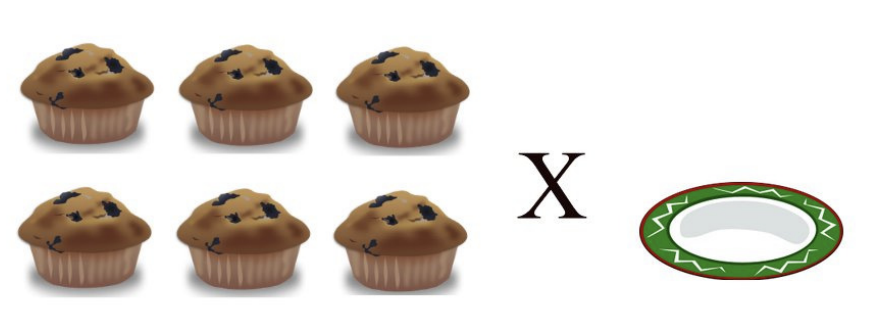# Multiplication Rules

Let's practice the multiplication rules we have learned in our lesson.
Language: English
Subject: Math > Multiplication
School grade:Canada
Multiplication Rules
4 x 10 = ____
Let's practice the multiplication rules we have learned in our lesson.
6 x 1 = ____ (remember our One Rule)
CHALLENGE QUESTION: What is 8123 x 1 = ____3 x 0 = ____
What is the answer to 8 x 10 = ____
9 x 1 = ___